## June 26, 2023

### Grothendieck–Galois–Brauer Theory (Part 1)

#### Posted by John BaezI’m slowly cooking up a big stew of ideas connecting Grothendieck’s Galois theory to the Brauer 3-group, the tenfold way, the foundations of quantum physics and more. The stew is not ready yet, but I’d like you to take a taste.

### Grothendieck’s Galois theory

In 1960 and 1961 Grothendieck developed a new approach to Galois theory in a series of seminars that got written up as SGA1. This is sometimes called Grothendieck’s Galois theory.

His approach was very geometrical. He treated the Galois group of a field $k$ as the fundamental group of a funny sort of ‘space’ associated to that field, called $Spec(k)$. This in turn let him treat sufficiently nice field extensions of $k$ as giving covering spaces of $Spec(k)$.

In short, he was exploiting the duality between commutative algebra and geometry, but pushing it further than ever before! An extension of fields is a map

$k \to k'$

from a field $k$ into a bigger field $k'$, but turning around the arrow here, he got a covering space

$Spec(k') \to Spec(k)$

Using this idea he was able to generalize Galois theory from fields to commutative rings and even more general things.

In the usual treatment of Grothendieck’s Galois theory the details get technical in ways that may be off-putting to people who aren’t committed to learning algebraic geometry. For example, what sort of ‘space’ is $Spec(k)$? It’s something called a ‘scheme’. How do we define a ‘covering space’ in this context? For that we need to know a bit about ‘Grothendieck topologies’. And so on. It seems we need to either hand-wave or dive into these topics. This is a pity because the underlying ideas are very simple — and among the most beautiful in 20th-century mathematics.

Luckily, a bunch of mathematicians thought about what Grothendieck had done and extracted some of its simple essence. That’s what I want to talk about here! So I won’t talk about algebraic geometry in any serious way, just simpler things.

But to get to these simpler things, it pays to look at a slight glitch in the story I’ve told so far.

I said that nice field extensions of a field $k$ give covering spaces of $Spec(k)$. But they can’t give all the covering spaces of $Spec(k)$, and it’s easy to see why. If we have two covering spaces of some space $X$ we can take their disjoint union and get another covering space. But what does that look like in in the world of algebra? If all covering spaces of $Spec(k)$ came from fields extending $k$, it would mean we could take the product of two fields extending a field $k$ and get another field extending $k$! You see, turning the arrows around in the definition of ‘disjoint union’ we get the definition of ‘product’.

But the product of fields is not usually a field! To include products of fields in our story, we need some more general commutative algebras. So we need to think about these.

They are called ‘commutative separable algebras’. And it turns out that commutative separable algebras over a field $k$ are exactly the same thing as finite products of ‘nice’ field extensions of $k$.

So, to understand Grothendieck’s approach to Galois theory in a simple low-brow way, it’s good to think about commutative separable algebras. But instead of starting by defining them, I want to make you see how inevitable this definition is. And for that, it’s easiest to look at a simpler context where this definition comes up. This will take a while, but it’s very nice math in its own right — and I’ll need it to make sense of the big stew of ideas that’s bubbling away in my brain.

There’s also a conjecture here, that you might help me prove.

### Sets versus vector spaces

Every set $X$ gives rise to complex vector space $F(X)$ whose basis is $X$. This is called the free vector space on $X$. Similarly, any function between sets $f \colon X \to Y$ gives a linear map between their free vector spaces, say $F(f) \colon F(X) \to F(Y)$, which sends basis elements to basis elements. We can summarize this, and a bit more, by saying there’s a functor

$F \colon \mathsf{Set} \to \mathsf{Vect}_{\mathbb{C}}$

This functor actually embeds the category of sets into the category of complex vector spaces. So it’s very natural to ask: if someone just hands you the category $\mathsf{Vect}_{\mathbb{C}}$, can you find the category $\mathsf{Set}$ sitting inside it?

Since every vector space has a basis, every object of $\mathsf{Vect}_{\mathbb{C}}$ is isomorphic to the free vector space on some set — so there’s nothing much to do there. But not every linear map $\ell \colon V \to W$ comes from a function between sets, no matter how you pick bases for $V$ and $W$. So there really is an interesting question here. It’s the question of how we find set theory lurking inside linear algebra. And this question will lead us inexorably to commutative separable algebras!

Since the category of sets has finite products, every set $X$ comes with a diagonal map

$\Delta_X \colon X \to X \times X$

and a unique map to the terminal set

$!_X \colon X \to 1$

I like to say that $\Delta_X$ duplicates elements of $X$, while $!_X$ deletes them. If we draw these maps using string diagrams, which we read from the top down, the diagonal $\Delta_X$ looks like this:

$\quad \qquad \qquad \qquad \qquad \qquad \qquad \qquad \qquad \qquad$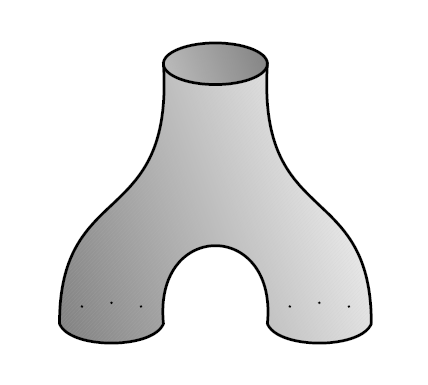while $!_X$ looks like this:

$\qquad \qquad \qquad \qquad \qquad \qquad \qquad \qquad \qquad \qquad$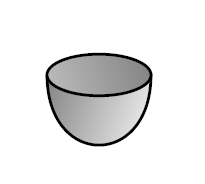If you duplicate an element of $X$ you can then duplicate either the first or the second copy, and the result is the same, so

$\qquad \qquad \qquad \qquad \qquad \qquad \qquad$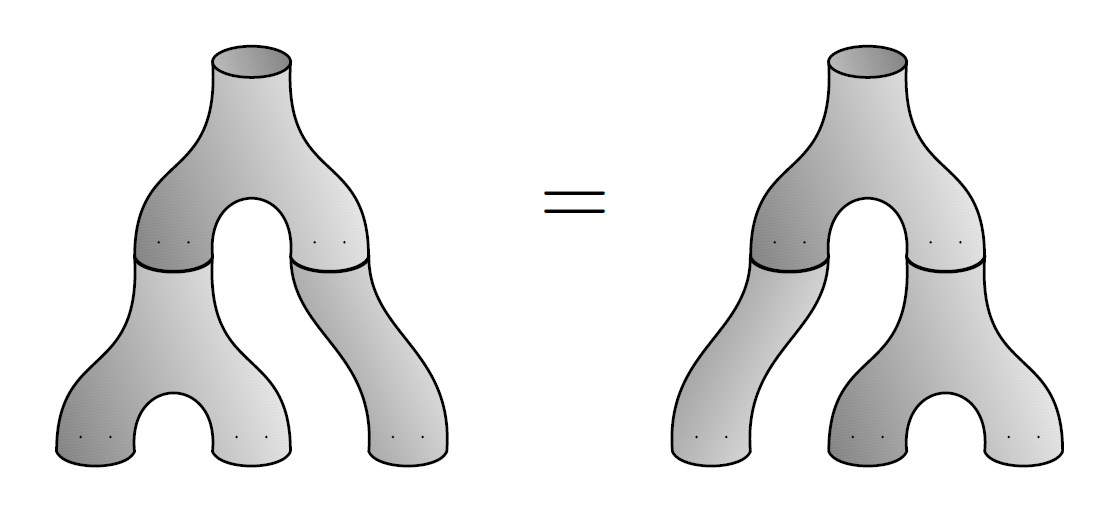Since this looks like an upside-down version of the associative law, this equation is called the coassociative law. Similarly, if you duplicate an element you can delete either the first or the second copy, and the result is the same as if you’d left that element alone:

$\qquad \qquad \qquad \qquad \qquad \qquad$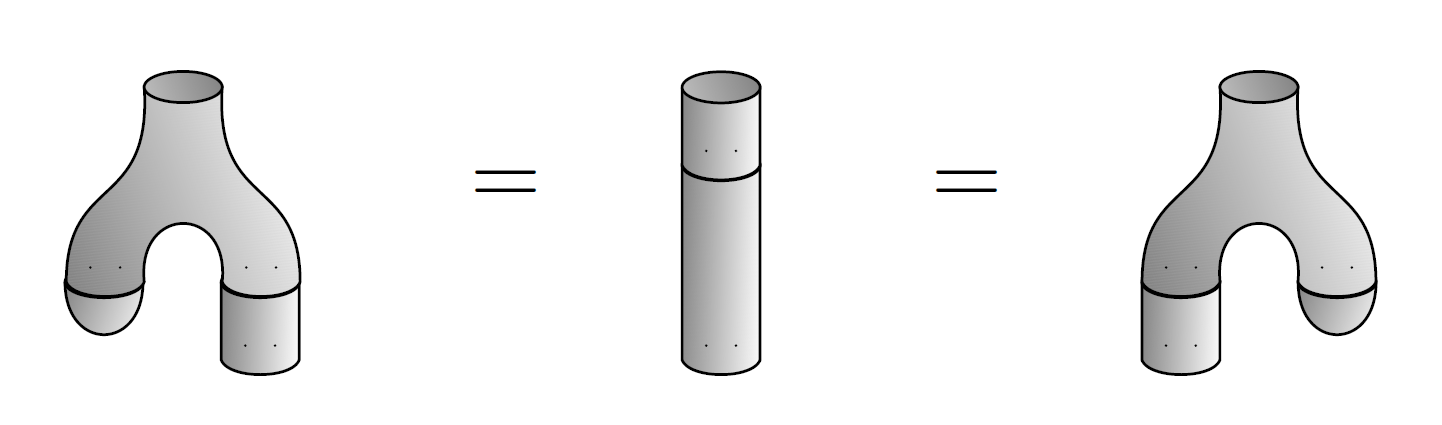These are called the counit laws.

Since all these laws are just the laws for a monoid written upside down, we call $(X,\Delta_X, !_X)$ a comonoid. Following this line of thought, we also call $\Delta_X$ the comultiplication and $!_X$ the counit of this comonoid.

Furthermore, if we duplicate an element of a set and then switch the two copies, it’s the same as if we had just duplicated but not switched:

$\qquad \qquad \qquad \qquad \qquad \qquad \qquad \qquad$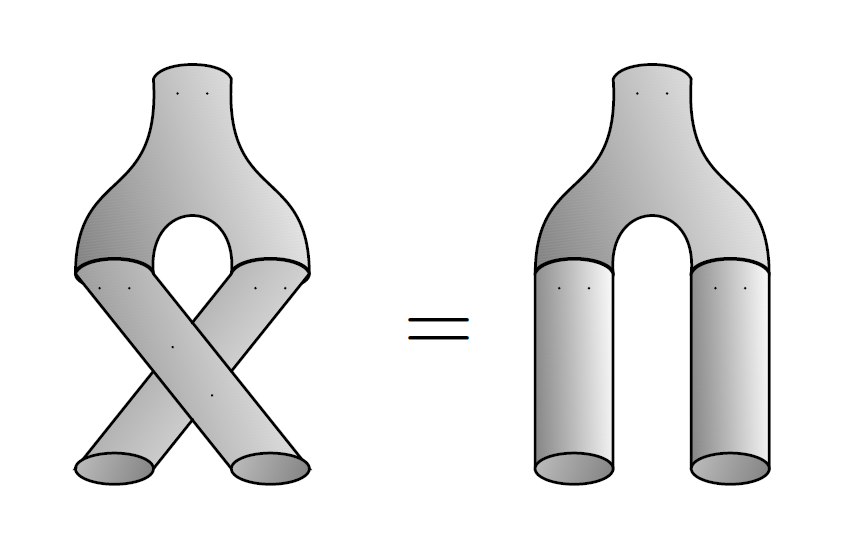This is called the cocommutative law.

So, all in all, we say every set is a cocommutative comonoid in $(\mathsf{Set}, \times, 1)$.

You can show more: for any category with finite products, every object is a cocommutative comonoid. Even better, it’s a cocommutative comonoid in a unique way! And better still, every morphism between objects is a comonoid homomorphism. That is: if you have a morphism $f \colon X \to Y$, it obeys

$\Delta_Y \circ f = (f \times f) \circ \Delta_X, \qquad !_Y \circ f = !_X$

These are fun equations to check if you know category theory. If that’s too hard, at least check them in the category $\mathsf{Set}$. And if that’s too hard, at least draw them in the style of the pictures I’ve been using here.

What does all this have to do with our problem: recognizing when a linear map between vector spaces comes from a function between sets? Well, the functor $F \colon \mathsf{Set} \to \mathsf{Vect}_{\mathbb{C}}$ turns cartesian products into tensor products:

$F(X \times Y) \cong F(X) \otimes F(Y)$

so we should expect that it sends any set, which is a cocommutative comonoid in $(\mathsf{Set}, \times, 1)$, to a cocommutative comonoid in $(\mathsf{Vect}_{\mathbb{C}}, \otimes, \mathbb{C})$. And this is indeed true! But we usually call such a thing a cocommutative coalgebra.

Let me say it again a different way. For any set $X$, the vector space $F(X)$ has a basis with one basis vector $e_i$ for each $i \in X$. It becomes a cocommutative coalgebra where the comultiplication

$\delta \colon V \to V \otimes V$

just duplicates each basis element:

$\delta(e_i) = e_i \otimes e_i$

and the counit

$\epsilon \colon V \to \mathbb{C}$

$\epsilon(e_i) = 1$

So, our ‘free vector space’ functor $F \colon \mathsf{Set} \to \mathsf{Vect}_{\mathbb{C}}$ sends sets to cocommutative coalgebras. Similarly, it sends functions between sets to coalgebra homomorphisms: linear maps between coalgebras that preserve the comultiplication and counit.

Even better, any coalgebra homomorphism $\alpha \colon F(X) \to F(Y)$ must equal $F(f)$ for some function $f \colon X \to Y$! This is a good thing to check, if you’re sinking under the weight of definitions and want to do something that forces you to understand them.

But there’s a fly in the ointment. Not every cocommutative coalgebra comes from a set in the way I’ve described! There are many others that are not even isomorphic to those coming from sets. For example, if you take any finite-dimensional commutative algebra, its dual vector space is a cocommutative coalgebra.

So, how can we tell which cocommutative coalgebras come from sets? There must be some way to characterize them. And indeed there is!

For starters, if $X$ is a set, we can think of the free vector space $F(X)$ as consisting of functions $f \colon X \to \mathbb{C}$ that are finitely supported: that is, nonzero at just finitely many points. And we can multiply two finitely supported functions in the usual pointwise way, and get another finitely supported function. So, $F(X)$ also has a multiplication

$m: F(X) \otimes F(X) \to F(X)$

which we can draw like this:

$\qquad \qquad \qquad \qquad \qquad \qquad \qquad \qquad \qquad \qquad$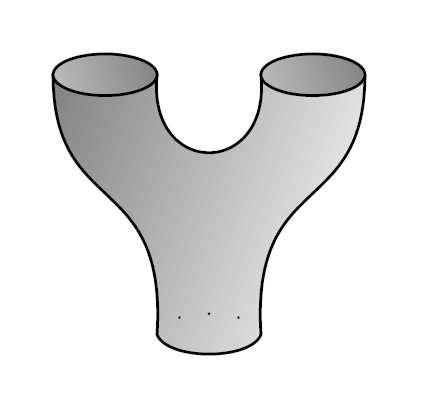Of course, this multiplication obeys the associative law:

$\qquad \qquad \qquad \qquad \qquad \qquad \qquad$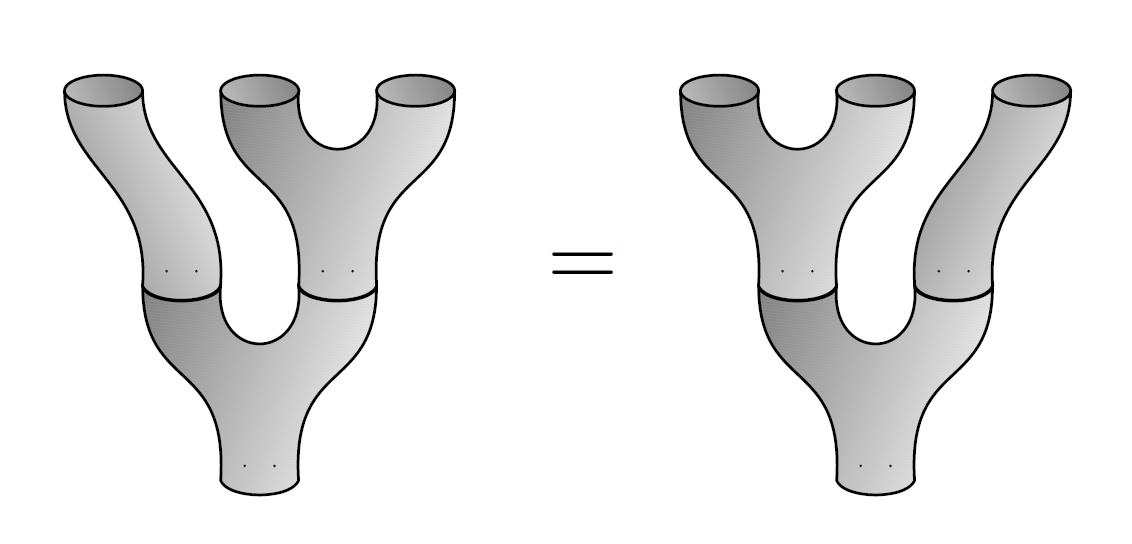and the commutative law:

$\qquad \qquad \qquad \qquad \qquad \qquad \qquad \qquad$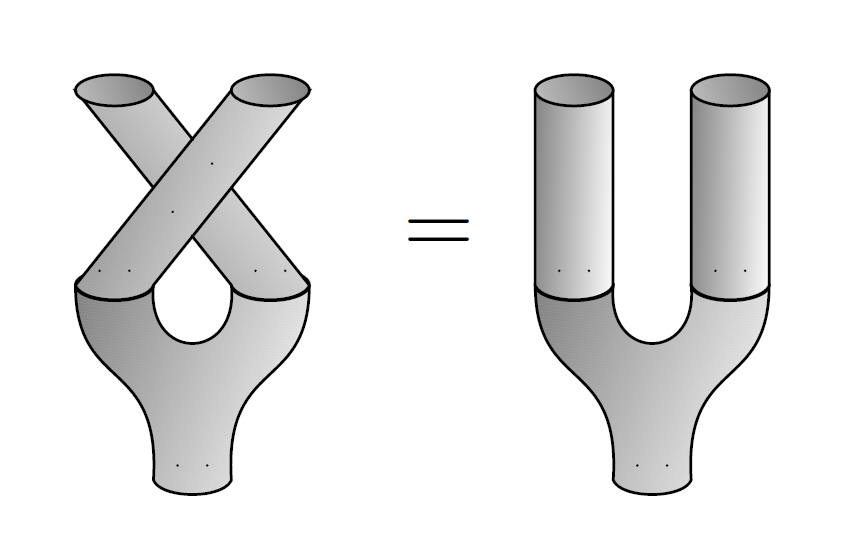It looks like we’re copying all our previous work, only upside-down! But there’s a catch. What about the unit? This should be a map

$i: \mathbb{C} \to F(X)$

so we’ll draw it like this:

$\qquad \qquad \qquad \qquad \qquad \qquad \qquad \qquad \qquad \qquad$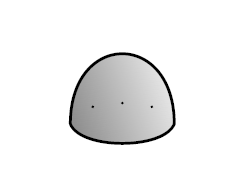And just as counit obeyed some counit laws, we’d like the unit to obey the unit laws:

$\qquad \qquad \qquad \qquad \qquad \qquad$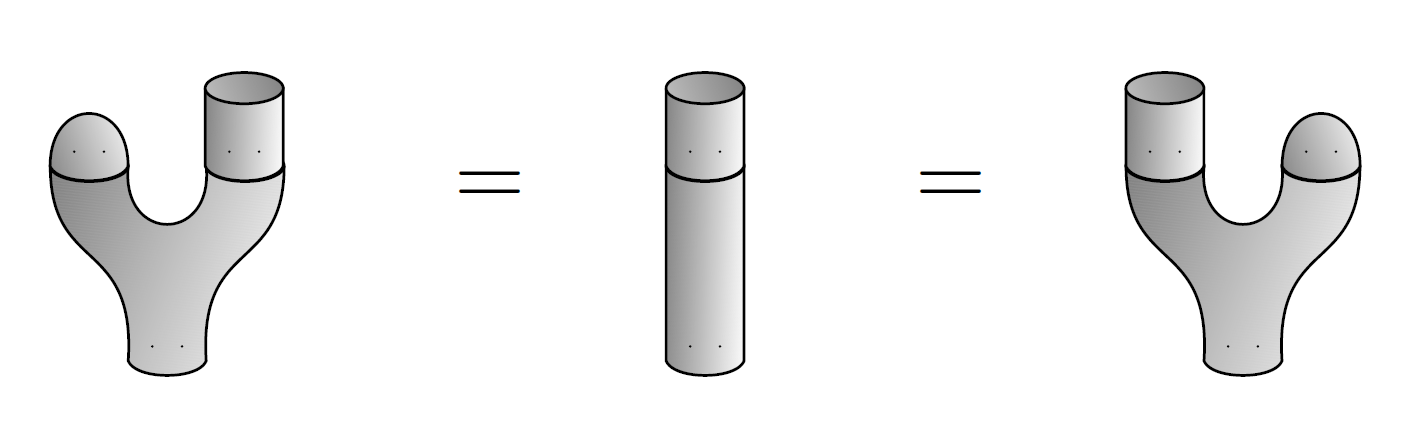If a vector space has a multiplication and unit obeying the associative, commutative and unit laws, it’s a commutative algebra. You probably knew that, if you’ve survived everything I’ve said so far.

But does such a unit actually exist for our vector space $F(X)$, equipped with the multiplication we gave it? If you think about it, the unit laws force $i(1) \in F(X)$ to be the constant function on $X$ that takes the value $1$ everywhere — since only that function acts as the unit for pointwise multiplication of functions. But remember: in the function picture, $F(X)$ consists of finitely supported functions! So we only get a unit when $X$ is a finite set!

So now we face a choice. We can either pursue our original goal of picking out the category sets and functions as a subcategory of $\mathsf{Vect}_{\mathbb{C}}$, or switch to a new goal: picking out the category of finite sets and functions.

Let’s switch to this new goal for a while. We can do it. And then — it will turn out — we can describe all sets and functions by dropping everything that involves the unit.

So far this is what we’ve seen: if $X$ is a finite set, the vector space $F(X)$ is both a commutative algebra and a cocommutative coalgebra.

But we’re not done yet, because the multiplication and comultiplication get along. That is, they obey some laws. First, they obey the Frobenius laws:

$\quad \qquad \qquad \qquad \qquad$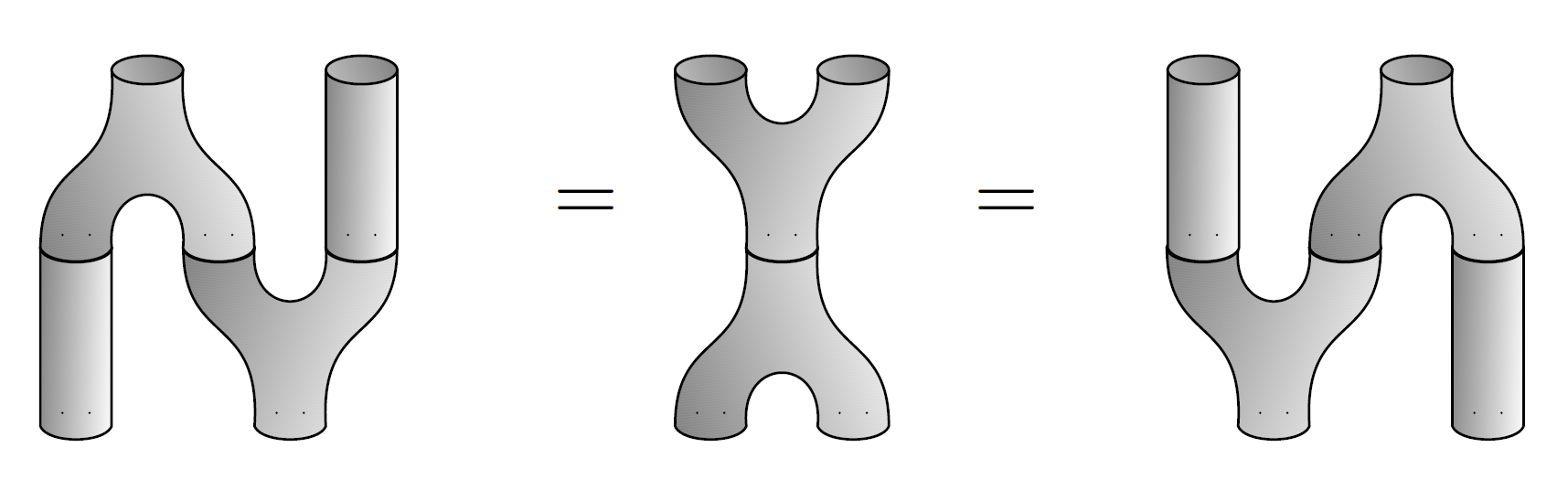Second, they obey the special law:

$\quad \qquad \qquad \qquad \qquad \qquad \qquad \qquad$I’ll let you check these things.

A vector space that’s both an algebra and coalgebra and also obeys the Frobenius laws is called a Frobenius algebra. If in addition it obeys the special law, it’s called a special Frobenius algebra. So, we’re seeing that the free vector space on a finite set is a commutative special Frobenius algebra. By the way, a Frobenius algebra is commutative if and only if it is cocommutative — so it’s enough to say “commutative”.

Whew! Are we done yet?

Yes and no. We are done with finding operations and laws. But in fact we’ve gone a bit too far — in a certain very interesting sense. We can define a Frobenius homomorphism of Frobenius algebra to be a map that preserves all the operations: multiplication, unit, comultiplication and counit. So, it’s both an algebra homomorphism and a coalgebra homomorphism. But then something shocking happens: all Frobenius homomorphisms are isomorphisms! There’s a fun proof using string diagrams, which I will let you reinvent.

In fact we can prove this:

Theorem 1. The category of commutative special Frobenius algebras in $\mathsf{Vect}_{\mathbb{C}}$, and Frobenius homomorphisms between them, is equivalent to the category of finite sets and bijections.

We were trying to find the category of sets and functions hiding in the world of linear algebra, but instead we found the category of finite sets and bijections. That’s okay. We just need to back off a bit to drop these restrictions. We can get rid of the bijection constraint like this:

Theorem 2. The category of commutative special Frobenius algebras in $\mathsf{Vect}_{\mathbb{C}}$, and coalgebra homomorphisms between these, is equivalent to the category of finite sets and functions.

To get a feeling for this, think about the unique function from a 2-element set to a 1-element set. Hitting this with our functor $F$ we get a linear map $\mathbb{C}^2 \to \mathbb{C}$. This map is just addition! Check that this map is not an algebra homomorphism, but is a coalgebra homomorphism.

Next let’s get rid of the finiteness constraint. I’ve already hinted at how: drop everything about the unit, since $F(X)$ doesn’t have a unit when $X$ is infinite.

To do this, we need a name for a commutative special Frobenius algebra that could be lacking a unit. Furthermore, to state a result like Theorem 2, we want to think about coalgebra homomorphisms between these things. So we’ll say this:

Definition. A coseparable coalgebra is a coalgebra for which there exists a multiplication that obeys the Frobenius and special laws.

Notice the subtlety: such a multiplication has to exist, but we don’t pick a specific one. So coseparability is a property of a coalgebra, not an extra structure.

All this led me to this guess, but I couldn’t prove it:

Conjecture 3. The category of cocommutative coseparable coalgebras in $\mathsf{Vect}_{\mathbb{C}}$, and coalgebra homomorphisms between these, is equivalent to the category of sets and functions.

Luckily, Theo Johnson–Freyd found a nice proof in the comments below. So we know how set theory sits inside the world of linear algebra! And we’d be done for the day if I were trying to explain coseparable coalgebras. But Grothendieck, like most algebraic geometers, preferred algebras to coalgebras — for good reasons, which I will not explain here.

So, let’s try to dream up an analogue of Conjecture 3 for algebras. Let’s copy our definition of coseparable coalgebra and say this:

Definition. A separable algebra is an algebra for which there exists a comultiplication that obeys the Frobenius and special laws.

This is the definition I’ve been leading up to all this time! And notice: now it’s the comultiplication whose existence is being treated as a mere property, not a structure that needs to be preserved. So, the correct maps between separable algebras are simply algebra homomorphisms.

Now we can state the theorem I’ve been leading up to all this time:

Theorem 4. The category of commutative separable algebras in $\mathsf{Vect}_{\mathbb{C}}$, and algebra homomorphisms between these, is equivalent to the opposite of the category of finite sets and functions.

Hey! Now we just get finite sets again! The reason is that any commutative separable algebra in $\mathsf{Vect}_{\mathbb{C}}$ is isomorphic to $F(X)$ for some set $X$, with the usual pointwise multiplication. But this set must be finite, for the algebra to have its unit.

This could be seen as a flaw if we were aiming to handle infinite sets. But if we want to do that, it seems we should use coalgebras. Grothendieck went with algebras.

### Conclusion

I’ve tried to make the theorems here plausible, but they are a bit harder to prove than you might think from what I wrote. In particular, they’re not true if we replace the complex numbers by the real numbers!

In particular, I said that every commutative separable algebra in $\mathsf{Vect}_{\mathbb{C}}$ is isomorphic to the algebra of complex-valued functions on a finite set. But the analogous thing fails in the real case:

Puzzle. Find a commutative separable algebra in $\mathsf{Vect}_{\mathbb{R}}$ that is not isomorphic to the algebra of real-valued functions on a finite set.

Perhaps unsurprisingly, this is connected to Galois theory. I’m talking about Grothendieck’s approach to Galois theory, after all! It turns out that the obvious analogues of Theorems 1, 2, and 4 hold for algebraically closed fields, but not other fields. Next time I’ll talk about what happens for other fields, though you can already guess from what I’ve said so far.

So, what I’ve really been discussing today is Grothendieck’s approach to Galois theory in the degenerate case of algebraically closed fields, where the Galois group is trivial. This is just the tip of the iceberg!

### References

Since the theorems I stated are a bit harder than they look, I should give you references to them.

This really digs into the meaning of separable algebras, and I owe a lot of my thoughts to this paper. However, these offer more traditional treatments:

• Frank DeMeyer and E. Ingraham, Separable Algebras Over Commutative Rings, Lecture Notes in Mathematics 181, Springer, Berlin, 1971.

• Timothy J. Ford, Separable Algebras, American Mathematical Society, Providence, Rhode Island, 2017.

To get proofs of what I’m calling Theorems 1, 2 and 4 out of these references, you have to scrabble around a bit. Ultimately it would be much nicer to find clean self-contained proofs, but for now let’s start with Theorem 4.5.7 in Ford’s book, which in my numbering system will be:

Theorem 5. Let $k$ be a field and $A$ a $k$-algebra. Then $A$ is a separable $k$-algebra if and only if $A$ is isomorphic to a finite direct sum of matrix rings $M_{n_i}(D_i)$ where each $D_i$ is a finite dimensional $k$-division algebra such that the center $Z(D_i)$ is a finite separable extension field of $k$.

Since we’re not doing Galois theory yet, let’s see what this says when $k$ is algebraically closed. Then the only division algebra over $k$ is $k$ itself, and the only finite separable extension field of $k$ is $k$ itself, so we get:

Theorem 6. Let $k$ be an algebraically closed field. Then an algebra in $\mathsf{Vect}_k$ is separable if and only if it is isomorphic to a finite product of matrix algebras over $k$.

(A finite product of algebras is the same as what ordinary people call a finite direct sum of algebras, but it is really the product in the category of algebras, not a coproduct.)

We’ll get interested in noncommutative separable algebras later in this series. But in the commutative case things simplify further:

Theorem 7. Let $k$ be an algebraically closed field. Then a commutative algebra in $\mathsf{Vect}_k$ is separable if and only if it is isomorphic to a finite product of copies of $k$.

Any such algebra, let’s call it $k^n$, can be given the structure of a special commutative Frobenius algebra by defining a comultiplication and counit in terms of the standard basis vectors $e_i \in k^n$ as follows:

$e_i \mapsto e_i \otimes e_i, \qquad e_i \mapsto 1$

Since any algebra homomorphism $\alpha \colon k^n \to k^m$ must send idempotents in $k^n$ to idempotents in $k^m$, it must send each sum of distinct basis vectors $e_i \in k^n$ to a sum of distinct basis vectors $f_j \in k^m$. It must also send the multiplicative identity of $k^n$, which is the sum of all the $e_i$, to the multiplicative identity in $k^m$, which is the sum of all the $f_j$. Thus we must have

$\alpha(e_i) = \sum_{j \in f^{-1}(i)} e_j$

for some function $f \colon \{1,\dots, m\} \to \{1, \dots, n\}$, and any function will do. This shows that the category of commutative separable algebras is equivalent to the opposite of the category of finite sets and functions! We get a slight strengthening of Theorem 4:

Theorem 4$\prime$. Let $k$ be an algebraically closed field. Then the category of commutative separable algebras in $\mathsf{Vect}_k$, and algebra homomorphisms between these, is equivalent to the opposite of the category of finite sets and functions.

We can also state this result in terms of Frobenius algebras, since we have:

Theorem 8. Let $k$ be any field. Then any separable algebra in $\mathsf{Vect}_k$ is finite-dimensional, and can be given a comultiplication and counit making it into a special Frobenius algebra.

The finite-dimensionality follows from Ford’s Theorem 4.5.7 above, and by definition any separable algebra can be given a comultiplication obeying the Frobenius and special laws, so the only news here is that we can then give it a counit obeying the counit laws. This is proved in the first theorem in Section 2 of Carboni’s paper.

This means that the category of (commutative) separable algebras is equivalent to the category of (commutative) special Frobenius algebras and algebra homomorphisms. So, we can restate Theorem 4$\prime$ in this way:

Theorem 4$\prime\prime$. Let $k$ be an algebraically closed field. Then the category of commutative special Frobenius algebras in $\mathsf{Vect}_k$, and algebra homomorphisms between these, is equivalent to the opposite of the category of finite sets and functions.

But it’s not good to say two categories are equivalent without specifying the equivalence, so let’s do that. There’s a functor

$k^- \colon \mathsf{FinSet}^{\mathrm{op}} \to \mathsf{FinVect}_k$

where $\mathsf{FinVect}_k$ is the category of finite-dimensional vector spaces over $k$. This functor maps each finite set $X$ to the vector space $k^X$ of $k$-valued functions on $X$. That vector space becomes an algebra with pointwise multiplication and a coalgebra with the comultiplication and counit we’ve seen:

$e_i \mapsto e_i \otimes e_i, \qquad e_i \mapsto 1$

and these fit together to form a commutative special Frobenius algebra. Putting everything together we get:

Theorem 4$\prime\prime\prime$. Let $k$ be an algebraically closed field. Then the functor

$k^- \colon \mathsf{FinSet}^{\mathrm{op}} \to \mathsf{FinVect}_k$

gives an equivalence between $\mathsf{FinSet}^{\mathrm{op}}$ and the category of commutative special Frobenius algebras and algebra homomorphisms between these.

Note the latter is equivalent to the category of commutative separable algebras.

We can get rid of the ‘opposite’ business using vector space duality: taking duals gives an equivalence between the category of finite-dimensional vector spaces and its opposite. Composing this with the above functor we get a functor

$(k^-)^\ast \colon \mathsf{FinSet}^{\mathrm{op}} \to \mathsf{FinVect}_k^{\mathrm{op}}$

sending any finite set $X$ to the dual of the vector space of $k$-valued functions on $X$. But this in turn gives a functor from $\mathsf{FinSet}$ to $\mathsf{FinVect}_k$, and that’s just our old friend the free vector space functor, restricted to finite sets:

$F \colon \mathsf{FinSet} \to \mathsf{FinVect}_k$

Taking the dual maps commutative special Frobenius algebras to commutative special Frobenius algebras, switching the multiplication and unit with the comultiplication and counit. Thus we get Theorem 3, which I’ll state more precisely now:

Theorem 3$\prime$. Let $k$ be an algebraically closed field. Then the functor

$F \colon \mathsf{FinSet} \to \mathsf{FinVect}_k$

gives an equivalence between $\mathsf{FinSet}$ and the category of commutative special Frobenius algebras and coalgebra homomorphisms between these.

Finally, let’s restrict $F$ to the category $\mathsf{FinBij}$ of finite sets and bijections. Clearly $F$ maps these to isomorphisms. But by my explicit description of all the algebra homomorphisms $\alpha \colon k^n \to k^m$, we see every isomorphism of these comes from a bijection $f \colon \{1,\dots, m\} \to \{1, \dots, n\}$. So, we get this more precise version of Theorem 1:

Theorem 1$\prime$. Let $k$ be an algebraically closed field. Then the functor

$F \colon \mathsf{FinSet} \to \mathsf{FinVect}_k$

gives an equivalence between $\mathsf{FinSet}$ and the category of commutative special Frobenius algebras and Frobenius algebra homomorphisms between these (which are all isomorphisms).

The fact that all homomorphisms of Frobenius algebras are isomorphisms is Lemma 2.4.5 here:

• Joachim Kock, Frobenius Algebras and 2D Topological Quantum Field Theories, Cambridge University Press, Cambridge 2004.
Posted at June 26, 2023 10:00 AM UTC

TrackBack URL for this Entry:   https://golem.ph.utexas.edu/cgi-bin/MT-3.0/dxy-tb.fcgi/3475

### Re: Grothendieck–Galois–Brauer Theory (Part 1)

My algebraic geometry is a bit rusty, but how come Spec(k) isn’t a trivial space with two points? After all Spec picks the prime ideals of a ring, and a field has only the improper ones (which I’m not even sure count)

Posted by: Matteo Capucci on June 26, 2023 10:15 PM | Permalink | Reply to this

### Re: Grothendieck–Galois–Brauer Theory (Part 1)

Uhm I guess Spec(k) has only one point (corresponding to the null ideal) and then the interesting part comes from the fact schemes are actually locally ringed spaces… Hence Spec(k) is basically k, since the functions over it’s only point are given by k

Posted by: Matteo Capucci on June 26, 2023 10:19 PM | Permalink | Reply to this

### Re: Grothendieck–Galois–Brauer Theory (Part 1)

Yes, the structure of $Spec(k)$ as a scheme is much more interesting than its structure as a mere topological space; this is one reason I called it a “funny sort of space”.

In particular, the étale topology (which is a Grothendieck topology on the category of schemes) allows us to define interesting covering spaces of $Spec(k)$, called étale covers, and any finite-degree separable field extension $k \to k'$ gives an étale cover $Spec(k') \to Spec(k)$. This then lets us define a fundamental group for $Spec(k)$, the étale fundamental group, which is related to these coverings in a manner that roughly mimics the usual theorem relating covering spaces of topological spaces to their fundamental groups.

However, I don’t plan to talk about any of this stuff in my series of posts! And you hinted at one reason: for any commutative ring $R$ (like the field you were just talking about) the scheme $Spec(R)$ is an affine scheme — and the category of affine schemes is simply defined to be the opposite of the category of commutative rings. So anything about affine schemes can be translated into the language of commutative rings, and that’s what I’ll try to do. This might get awkward if I were mainly going to talk about algebraic geometry, but in fact I’ll be sailing off in a number of other directions.

Posted by: John Baez on June 27, 2023 12:19 AM | Permalink | Reply to this

### Re: Grothendieck–Galois–Brauer Theory (Part 1)

“It turns out that the obvious analogues of Theorems 1, 2, and 4 hold for algebraically closed fields, but not other fields.”

Admit it: you set this up to come out 1,2,4 intentionally. I am curious to see Theorem 8.

I am happy that you have “(co)separable” as a property rather than a structure, as the choice of such should make them “(co)separated”. It bugs me when people talk about spin manifolds – are they spinnable, or are they spun?

Posted by: Allen Knutson on June 27, 2023 2:05 AM | Permalink | Reply to this

### Re: Grothendieck–Galois–Brauer Theory (Part 1)

I did not set it up intentionally. Initially I thought Conjecture 3, classifying coseparable coalgebras, was known. Now I really want someone to prove it! There are a few papers on coseparable coalgebras, with some free ones including:

There are others, which sometimes have the feel of being written by people who have nothing better to do than take known results and stick ‘co’ in front of everything. Like this title: “Cosemisimple coalgebras and coseparable coalgebras over coalgebras”. Sort of like taking results about left modules and publishing analogous results for right modules!

But tracing back the line of papers to its source:

I see, suddenly, that he states an offhand remark that would imply Conjecture 3:

[…] it can be shown that a coalgebra $C$ is coseparable if and only if $C$ is the direct sum of a collection of finite-dimensional coalgebras, each of which is the dual coalgebra to a finite-dimensional separable algebra.

And more, since this is working over an arbitrary field, not necessarily algebraically closed! Since separable algebras (necessarily finite-dimensional) over a field have been classified, this does the job. It would be sad if this result never received a proper proof.

I have seen ‘spun honey’ for sale in stores. Do they sell ‘spinnable honey’ for the DIY crowd?

Posted by: John Baez on June 27, 2023 4:59 PM | Permalink | Reply to this

### Re: Grothendieck–Galois–Brauer Theory (Part 1)

Spotted a typo: “among the most beautifufl”. And somewhere else: “Even better, it’s a cocommutative monoid in a unique way way!” Should this be a “comonoid” here?

It looks intriguing. Could you send my compliments to the chef?

Posted by: Stéphane Desarzens on June 27, 2023 6:54 AM | Permalink | Reply to this

### Re: Grothendieck–Galois–Brauer Theory (Part 1)

Thanks for catching all those typos! I’ve fixed them. It’s funny how I can reread something I’ve written dozens of times, improving it here and there, and still not see such glaring mistakes.

I’ll pass your compliments on to the chef.

Posted by: John Baez on June 27, 2023 4:29 PM | Permalink | Reply to this

### Re: Grothendieck–Galois–Brauer Theory (Part 1)

It turns out that a cogroup object in the category of groups is the same thing as a pointed set. And from pointed sets you can recover ordinary sets.

It’s interesting that the construction for complex vector spaces also involves some internal comonoid.

I chose the category of groups in that question because I thought it would be a representative example of other categories of algebraic objects. But in fact I haven’t been able to recover sets from any category of modules. The coalgebra construction isn’t enough because I don’t even know how to define tensor product in terms of first-order statements about the category.

Posted by: Oscar Cunningham on June 27, 2023 7:44 PM | Permalink | Reply to this

### Re: Grothendieck–Galois–Brauer Theory (Part 1)

Whenever you have a forgetful functor $U : \mathsf{C} \to \mathsf{Set}$ that has a left adjoint $F: \mathsf{Set} \to \mathsf{C}$, the objects $F(X) \in \mathsf{C}$ coming from sets will come with maps

$F(\Delta_X) : F(X) \to F(X \times X)$

$F(!_X) : F(1) \to F(X)$

that make $X$ into something resembling a cocommutative comonoid.

However we need a bit more, like $F$ being oplax monoidal with respect to some tensor product $\otimes$ on $\mathsf{C}$, to get morphisms

$F(X) \to F(X) \otimes F(X)$

$1 \to F(X)$

making $F(X)$ into an honest cocommutative comonoid internal to $(C,\otimes)$. When $\mathsf{C}$ is the category of modules of a commutative ring this works with the usual tensor product of modules, and that’s what I was exploiting for vector spaces. Something similar would work for abelian groups… but groups behave in a more tricky way.

The category of vector spaces (over some fixed field) is a bit unusual among these examples, in that every vector space is free on some set… well, at least if you use the axiom of choice. That doesn’t happen a lot:

Posted by: John Baez on June 27, 2023 8:00 PM | Permalink | Reply to this

### Re: Grothendieck–Galois–Brauer Theory (Part 1)

I’m still thinking about your puzzle. Maybe the complex numbers are separable with a comultiplication that sends a+bi to (a/2, b/2, b/2, -a/2)?

Another interesting example of a nonfree Frobenius algebra exists in the category of bundles on the Klein bottle. Usually when you try to equip the tangent bundle with a global basis it fails because the two basis elements swap places as you go around the bottle. But you only need an ‘unordered basis’ to specify a Frobenius algebra, so this doesn’t cause a problem.

Posted by: Oscar Cunningham on June 27, 2023 9:56 PM | Permalink | Reply to this

### Re: Grothendieck–Galois–Brauer Theory (Part 1)

You are definitely on the right track with that separable algebra puzzle, though I can’t quickly check that your comultiplication

$\delta \colon \mathbb{C} \to \mathbb{C} \otimes_\mathbb{R} \mathbb{C} \quad (\cong \mathbb{C} \oplus \mathbb{C})$

obeys the necessary rules: coassociativity, the Frobenius laws, and the special law. There are ways to set things up so that some of these, at least, are easy to check.

Another interesting example of a nonfree Frobenius algebra exists in the category of bundles on the Klein bottle. Usually when you try to equip the tangent bundle with a global basis it fails because the two basis elements swap places as you go around the bottle. But you only need an ‘unordered basis’ to specify a Frobenius algebra, so this doesn’t cause a problem.

Yes, this is very nice! Categories of vector bundles on spaces provide a really interesting context for studying ‘twisted’ separable algebras, and I’ll probably get into that a bit more later, since in a way this is what ‘Brauer groups’ are all about.

Posted by: John Baez on June 27, 2023 11:27 PM | Permalink | Reply to this

### Re: Grothendieck–Galois–Brauer Theory (Part 1)

There are ways to set things up so that some of these, at least, are easy to check.

Ah, I see it now. Define a real-bilinear form on $\mathbb{C}$ by $x,y\mapsto \mathrm{Re}(x y)$. This has signature $(+1,-1)$ and so is nondegenerate. In diagrammatic terms this means that we can now bend strings from upward to downward, or vice versa.

The remaining important observation is that $\mathrm{Re}((x y)z)=\mathrm{Re}(x(y z))$. This means that the multiplication remains the same even if we swap an input with an output.

So we can define the comultiplication by taking the multiplication and bending one of the inputs to make an output. All the diagrams we are required to show can be dealt with by rearranging them into diagrams about multiplication.

Posted by: Oscar Cunningham on June 28, 2023 8:58 AM | Permalink | Reply to this

### Re: Grothendieck–Galois–Brauer Theory (Part 1)

Wow, I considered giving you a hint but you didn’t need it! Here’s the hint, expanded a bit, for those who don’t get what you just did.

Suppose have a finite-dimensional algebra $A$ over some field $k$ and you want to enhance it to a Frobenius algebra. Then it’s sufficient (and necessary) to find a nondegenerate bilinear form $\beta \colon A \times A \to k$ that is ‘associative’:

$\beta( a b , c ) = \beta( a , b c)$

This then determines a vector space isomorphism $\sharp \colon A \to A^\ast$ by

$\sharp(a) (b) = \beta(a, b)$

which in turn lets you dualize the multiplication and unit to give $A$ a comultiplication and counit. The ‘associative’ law for $\beta$ implies the associativity of the comultiplication and also the Frobenius law; the counit laws follow from the unit laws.

If you go about things this way, and you’re hoping to get a special Frobenius algebra (and thus a separable algebra), you still have to check that your comultiplication and multiplication obey the ‘special’ law: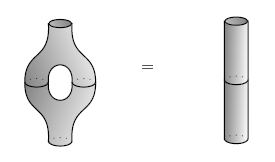I’m not sure your proposed $\beta$ makes this law hold, but if not, I think you can just rescale it to get that law to hold.

Posted by: John Baez on June 28, 2023 8:39 PM | Permalink | Reply to this

### Re: Grothendieck–Galois–Brauer Theory (Part 1)

You asked whether a coseparable coalgebra (over an algebraically closed field) is merely a set. Here “coseparable coalgebra” in particular means cocommutative.

The answer is yes, and most likely due to Sweedler. Some notation: given a symmetric monoidal category $\mathcal{V}$, I’ll write $Cog(\mathcal{V})$ for the category of cocommutative coalgebras in $V$. I’ll also write “Ind” for the ind objects. Here are some basic facts. The facts for Vec hold over any field:

$Ind(FinSet) = Set$ $Ind(FinVec) = Vec$ $Ind(Cog(FinVec)) = Cog(Ind(FinVec)).$

In particular, every coalgebra is the filtered union of its finite-dimensional subalgebras. So to understand $Cog(Vec)$ it suffices to understand $Cog(FinVec)$.

Lemma: If $C \in Cog(Vec)$ is coseparable, then all of its subcoalgebras are.

Proof: The frobenius axiom is checked locally, so it suffices to show that a subcoalgebra is closed under multiplication. Which is obvious from taking a frobenius axiom and sticking a counit on one of the outputs.

Let me write $CosepCog \subset Cog$ for the full subcategory on the coseparables.

$CosepCog(FinVec_{\mathbb{k}}) = Gal(\mathbb{k}^sep/\mathbb{k})-FinSet$

is the category of sets with an action by the absolute Galois group.

You know that taking any Gal-set and linearizing it supplies a coseparable coalgebra. The lemma tells you that you get the other direction. So you get the whole proof.

Here is a neat general fact. Let $\mathcal{C}$ be a symmetric closed-monoidal locally presentable category. Then $Cog(\mathcal{C})$ is Cartesian-closed locally presentable. (The cartesian product of cocommutative coalgebras is their tensor product.) Moreover, $Forget: Cog(\mathcal{C}) \to \mathcal{C}$ is cocontinuous and symmetric monoidal. Indeed:

Fact: $Cog(\mathcal{C}) \to \mathcal{C}$ is the universal Cartesian-closed symmetric monoidal locally presentable category mapping cocontinuously and symmetric monoidally to $\mathcal{C}$.

I won’t prove this fact here. In general, and in particular in the Vec case, $Cog(\mathcal{C})$ is not quite a topos. But it is very close to one.

Since $Forget$ is cocontinuous between locally presentable categories, it is a left adjoint. Its right adjoint deserves the name “cofree”. It is not a symmetric coalgebra: it is much bigger than that.

The way you should think about an element of $Cog(Vec)$ is that it is like a Gal-set, but every orbit (which automatically carries a field) also has a fuzzy infinitesimal neighbourhood (which could be a field extension). Over an algebraically closed field $\bar{\mathbb{k}}$, the cofree coalgebra is built from a vector space $V$ by taking every point in $V$, and giving it an infinitesimal neighbourhood isomorphic to $V$. Notice that the number of points depends on the cardinality of $\bar{\mathbb{k}}$: Cofree does not commute with transcendental extensions. Over a perfect but nonalgebraically closed field, for finite-dimensional vector spaces you take every closed point in $Spec(Sym(V*))$. In other words, these are the “points” of V where the point might be described by a field extension. I’ll let you enjoy the imperfect case.

Geometrically, you should think of the elements of $Cog(Vec)$ as being the geometric objects — they are the “sets” or “spaces” — and “Forget” is really the “linearization” map that takes a set to the vector space of distributions on that set. The reason elements of $Cog(Vec)$ look very disconnected is because we’re only seeing the topology that’s seen by distributions.

Posted by: Theo Johnson-Freyd on June 28, 2023 3:29 PM | Permalink | Reply to this

### Re: Grothendieck–Galois–Brauer Theory (Part 1)

Thanks! I knew that any coalgebra $A$ is the union of its finite-dimensional sub-coalgebras, but I couldn’t see why those would be closed under the chosen multiplication $\mu \colon A \otimes A \to A$, so that’s where I got stuck. For those who don’t get it: if we take the Frobenius lawsand cap off the left-hand output at the bottom using the counit, we get multiplication in the middle, and two other ways to write multiplication. The right-hand way expresses it as

$A \otimes A \xrightarrow{1 \otimes \delta} A \otimes A \otimes A \xrightarrow{\epsilon \circ \mu\, \otimes \,1} k \otimes A \cong A$

where $k$ is our field, $\delta$ is the comultiplication and $\epsilon$ is the counit. Regardless of what $\epsilon \circ \mu$ is, we see that if $B \subseteq A$ is a sub-coalgebra, multiplication restricts to a map

$B \otimes B \xrightarrow{1 \otimes \delta} A \otimes A \otimes A \xrightarrow{\epsilon \circ \mu\, \otimes \,1} k \otimes A \cong A$

whose image lies in $B$, since $\delta$ maps $B$ to $B \otimes B$.

I’ll respond to your other points later — thanks!

Posted by: John Baez on June 28, 2023 6:51 PM | Permalink | Reply to this

### Re: Grothendieck–Galois–Brauer Theory (Part 1)

Before I forget: there’s a discussion of coseparable coalgebras here:

This studies a version of the Brauer group for a cocommutative coalgebra $R$, whose elements are equivalence classes of ‘Azumaya coalgebras’ over $R$. It should probably be a special case of Niles Johnson’s setup, which lets us define a Brauer group for any compact closed bicategory, e.g. the bicategory of

• cocommutative coalgebras over $R$,
• bi-comodules,
• bi-comodule homomorphisms.
Posted by: John Baez on June 30, 2023 7:10 AM | Permalink | Reply to this

### Mozibur Rahman

I didn’t get Galois theory and couldn’t get excited about it until I came the book, Galois Theories by Borceaux & Janelidze. They cover Grothendieck’s Galois theory and give a categorical generalisation. The details are hazy now since its been around ten years since I first looked at it.

One other point which few people seem to mention is that although Galois showed that there was no general formula for the roots of a polynomial by radicals this does not necessarily mean there is no such formula if we upgrade our toolkit from radicals to something more fancy. And it turns out upgrading to modular functions is enough as shown by Jordan with explicit formulae given by Thomae. Umemura rewrote these using higher theta functions. This is one good reason to be interested in modular and theta functions. I learnt all this from Wikipedia.

Posted by: Mozibur Rahman Ullah on June 30, 2023 5:26 AM | Permalink | Reply to this

### Mozibur Rahman

There are others, which sometimes have the feel of being written by people who have nothing better to do than take known results and stick ‘co’ in front of everything.

Yeah, it’s a cop-out!

Geddit?

Posted by: Mozibur Rahman Ullah on June 30, 2023 9:23 AM | Permalink | Reply to this

### Re: Mozibur Rahman

When I’m feeling sad and my wife gives me a pout, I tell her that’s a copout.

Posted by: John Baez on June 30, 2023 7:39 PM | Permalink | Reply to this

### Harry

So am I right that $\mathbb{C}$ is an answer to the puzzle because -1 has a root, which doesn’t happen when you have pointwise multiplication of functions $2 \rightarrow \mathbb{R}$? Ultimately I guess this is because $Gal (\mathbb{C} / \mathbb{R}) = \mathbb{Z}_2$:does this mean complex conjugation is related to an acceptable comultiplication?

Posted by: Harry Wilson on July 1, 2023 4:56 PM | Permalink | Reply to this

### Re: Harry

Yes, $\mathbb{C}$ is a great example of commutative separable algebra over $\mathbb{R}$ that’s not the algebra of real-valued functions on a finite set! Oscar Cunningham worked out some of the details in an earlier comment here.

I think what matters is not so much complex conjugation directly as that $\mathbb{C}$ has solutions of polynomial equations that don’t have solutions in $\mathbb{R}$.

This subject gets a lot richer when we consider separable algebras over $\mathbb{Q}$, because then there are lots of polynomial equations lacking solutions, which give lots of commutative separable algebras that aren’t just algebras of $\mathbb{Q}$-valued functions on finite sets. Albert, Brauer, Hasse and Noether worked out the details, and I’ve been trying to learn about this. I should explain some of this stuff sometime.

Posted by: John Baez on July 1, 2023 6:46 PM | Permalink | Reply to this
Read the post Grothendieck--Galois--Brauer Theory (Part 2)
Weblog: The n-Category Café
Excerpt: The fundamental theorem of Grothendieck's Galois theory.
Tracked: August 25, 2023 3:35 PM

Post a New Comment